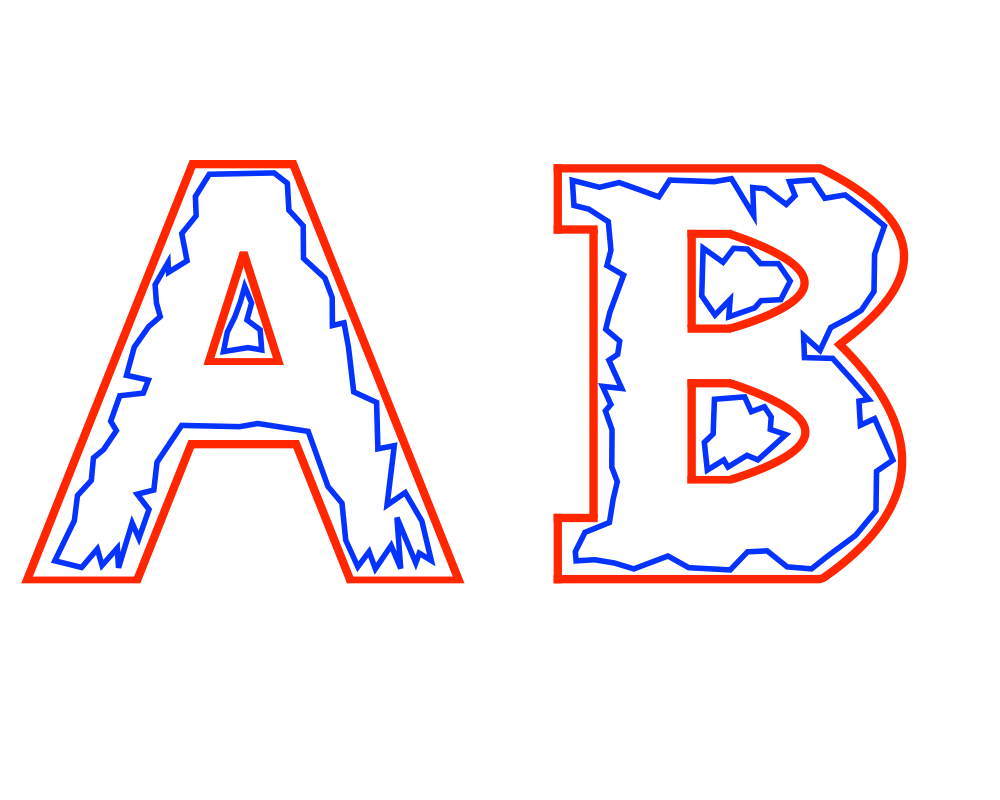# Simplifying PolygonsInputOutput

Input Description: A polygon or polyhedron $$p$$, with $$n$$ vertices.
Problem: Find a polygon or polyhedron $$p'$$ with $$n'$$ vertices, where the shape of $$p'$$ is close to $$p$$ while $$n' << n$$.

Excerpt from The Algorithm Design Manual: Polygon simplification has two primary applications. The first is in cleaning up a noisy representation of a polygon, perhaps obtained by scanning a picture of an object. By processing it, we hope to remove the noise and reconstruct the original object. The second is in data compression, where given a large and complicated object, we seek to simplify it by reducing detail. Ideally, we obtain a polygon with far fewer vertices that looks essentially the same. This can be a big win in computer graphics, where replacing a large model with a smaller model might have little visual impact but be significantly faster to render.

Go To Main Page